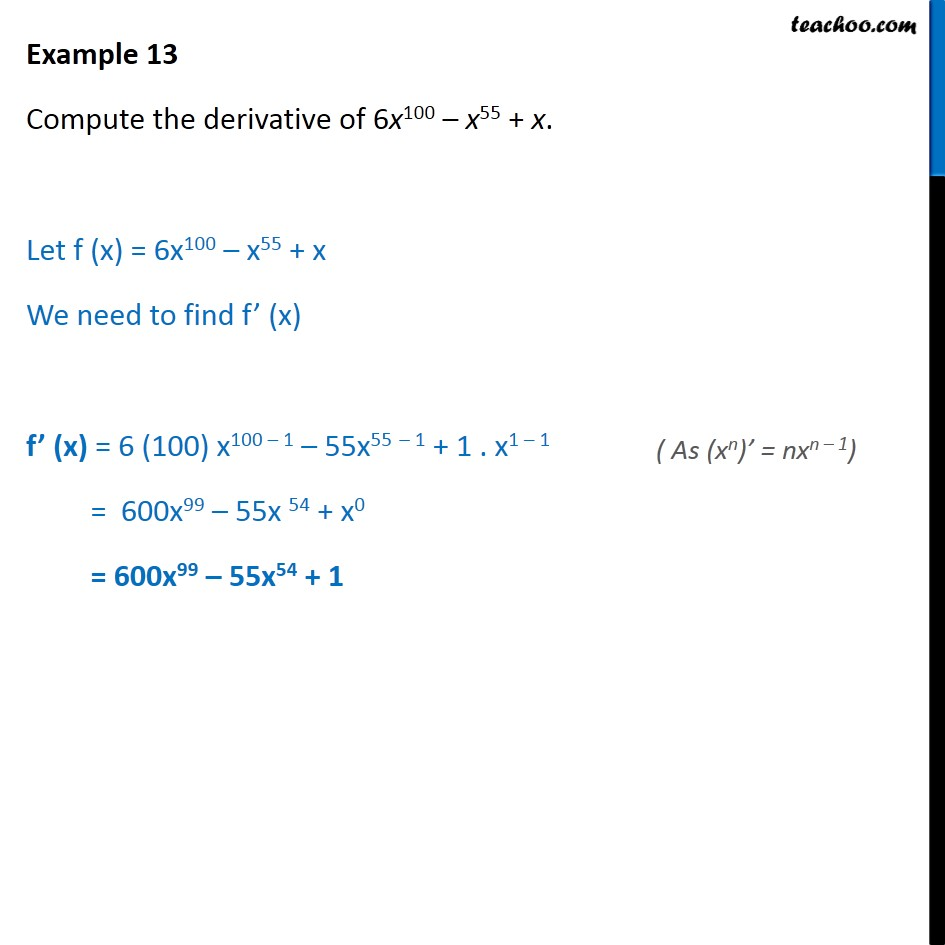Subscribe to our Youtube Channel - https://you.tube/teachoo

1. Chapter 13 Class 11 Limits and Derivatives
2. Serial order wise
3. Examples

Transcript

Example 13 Compute the derivative of 6x100 x55 + x. Let f (x) = 6x100 x55 + x We need to find f (x) f (x) = 6 (100) x100 1 55x55 1 + 1 . x1 1 = 600x99 55x 54 + x0 = 600x99 55x54 + 1

Examples